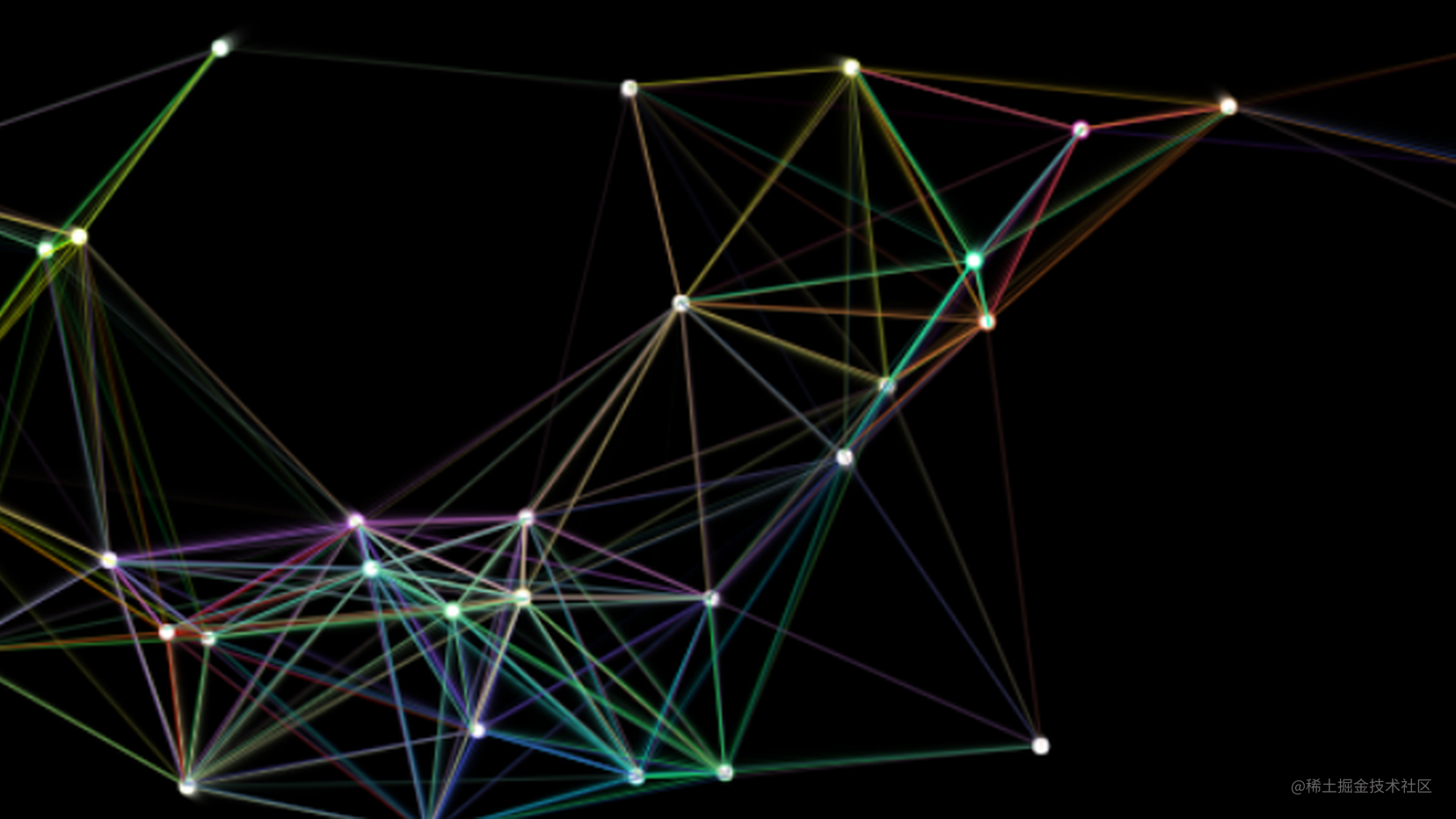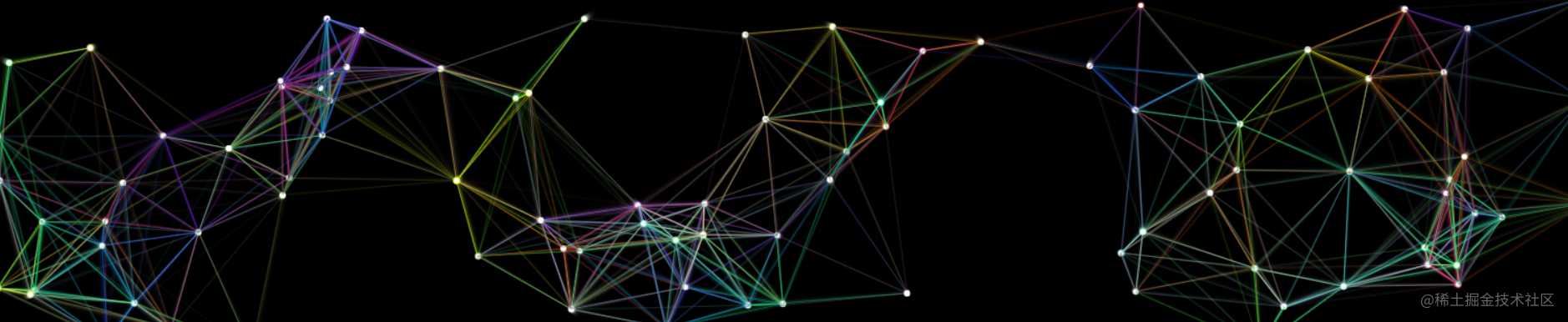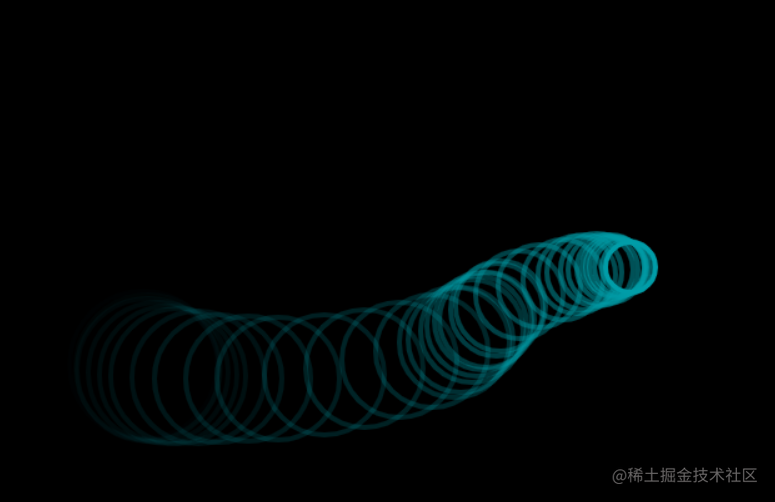# canvas实现粒子动画效果## canvas 粒子连线动效

wasm 实现：github.com/kiliaosi/wa…

wasm 实现使用的是 rust 语言，实现过程很丝滑~

## 主要逻辑简述

1. 准备一个空白的画布。

2. 将一定数量的圆形图形分布到画布的随机位置。

3. 给小球赋予初始的移动方向（x 轴及 Y 轴），并且给定轴方向的速度（简单起见，这里的速度在整个程序周期内，不会发生变化）。

4. 使用 setInterval 或者 requestAnimationFrame（推荐）来实现动画。

5. 浏览器四边的碰撞检测（碰撞之后需要改变小球的移动方向，避免小球移出画布）。

经过上述步骤后，读者应该能实现一个简单的无线条的粒子动效。

6. 粒子连线

• 连线的实现逻辑也比较简单，就是计算当前小球和其他小球在直角坐标系中的一个距离（需要借助三角函数），将距离限度内的小球连线，线条颜色使用 rgba 调色，这样一来会实现远近距离的线条的透明度不同。

### 第一阶段准备一个画布并初始化

• 新建目录，新建 index.html、canvas.js 文件

• html 初始源码

html 部分只需要如下代码就可以，后续也不会再有改动

``````<!DOCTYPE html>
<html lang="en">
<meta charset="UTF-8" />
<meta http-equiv="X-UA-Compatible" content="IE=edge" />
<meta name="viewport" content="width=device-width, initial-scale=1.0" />
<title>Document</title>
<body>
<canvas id="myCanvas" width=""></canvas>
<script src="./canvas.js"></script>
</body>
</html>
``````
• js

js 初始源码源码如下

1. 获取 canvas 标签
2. 初始化 canvas 的宽高
3. 获取 canvas 上下文（画笔）
``````// 获取canvas标签
let canvas = document.getElementById("myCanvas");

// 初始化画布的宽高，根据浏览器窗口的宽高来初始化,并且保存浏览器宽高（这里主要是为了后续在浏览器边缘的碰撞检测处使用）
let width = (canvas.width = window.innerWidth);
let height = (canvas.height = window.innerHeight);

// 获取canvas上下文（画笔）
let ctx = canvas.getContext("2d");
``````

### 工具函数

1. ramdom 函数主要功能为在给定的范围内生成一个随机数的函数。
2. randomColor 函数主要功能为使用 random 函数，生成随机的一个颜色。
``````function random(min, max) {
let num = Math.floor(Math.random() * (max - min) + min);
if (num === 0) {
num = 1;
}
return num;
}
// 随机生成颜色 （rgb）
function randomColor() {
return `rgb(\${random(0, 255)},\${random(0, 255)},\${random(0, 255)})`;
}
``````

### 实现小球类

``````/**
* @param {number} x 横坐标
* @param {number} y 纵坐标
* @param {number} vx 沿横轴方向的速度 （可以为负数）
* @param {number} vy 沿纵轴方向的速度（可为负数）
* @param {number} size 小球的半径
* @param {string} color 颜色
* @param {string} line 线条颜色
*
*/

function Ball(x, y, vx, vy, size, color, line) {
this.x = x;
this.y = y;
this.vx = vx;
this.vy = vy;
this.size = size;
(this.color = color), (this.lineColor = line);
}
``````

### 绘制当前小球对象的方法

``````Ball.prototype.draw = function () {
// 开始绘制（路径）
ctx.beginPath();
// 设置画笔的填充颜色
ctx.fillStyle = this.color;

// 根据坐标绘制小球
ctx.arc(this.x, this.y, this.size, 0, 2 * Math.PI);

// 填充
ctx.fill();
};
``````

### 更新小球的状态，让小球动起来

``````Ball.prototype.update = function () {
// 检查当前小球的X坐标加上当前的小球半径是否大于浏览器宽度（右边缘碰撞检测）
// 检查当前小球的X坐标加上当前的小球半径是否小于0（左边缘碰撞检测）
// 上述的两个条件如果满足其中一个，就需要调转方向（X轴速度vx设置为当前vx的相反数）
if (this.x + this.size >= width || this.x - this.size <= 0) {
this.vx = -this.vx;
}

// 检查当前小球的Y坐标加上当前的小球半径是否大于浏览器高度（下边缘碰撞检测）
// 检查当前小球的Y坐标加上当前的小球半径是否小于0（上边缘碰撞检测）
// 上述的两个条件如果满足其中一个，就需要调转方向（Y轴速度vy设置为当前vy的相反数）
if (this.y + this.size >= height || this.y - this.size <= 0) {
this.vy = -this.vy;
}

// 根据速度值改动小球的坐标
this.x += this.vx;
this.y += this.vy;
};
``````

### 生成小球对象列表

``````let list = [];
for (let i = 0; i <= 90; i++) {
let circle = new Ball(
random(0, width),
random(0, height),
random(-6, 6) * (1 / 3.0),
random(-6, 6) * (1 / 3.0),
3,
"rgb(255,255,255)",
`rgba(\${random(0, 255)},\${random(0, 255)},\${random(0, 255)}`
);
list.push(circle);
}
``````

### 循环函数

``````function loopCircle() {
// 刷新画布
ctx.fillStyle = "rgba(0,0,0,0.6)";
ctx.fillRect(0, 0, width, height);

// 双重循环
// 这里主要是为了计算小球之间的一个二维空间距离，性能不高，有很大的优化空间，读者有兴趣可以自行优化
for (let i = 0; i < list.length; i++) {
for (let j = 0; j < list.length; j++) {
// 计算当前小球的距离
let lx = list[j].x - list[i].x;
let ly = list[j].y - list[i].y;
let LL = Math.sqrt(Math.pow(lx, 2) + Math.pow(ly, 2));

//比对：当距离满足时，绘制线条，以rgba实现过渡
if (LL <= 180) {
ctx.beginPath();

// 这里补足了前面的半截rgba线条颜色
ctx.strokeStyle = `\${list[i].lineColor},\${(180 - LL) / 180})`;

// 绘制线条
ctx.moveTo(list[i].x, list[i].y);
ctx.lineWidth = 1;
ctx.lineTo(list[j].x, list[j].y);
ctx.stroke();
}
}

// 绘制小球
list[i].draw();

// 更新坐标
list[i].update();
}

// 执行动画（这里传入函数自身后，该回调函数会在浏览器下一次重绘之前再次执行）
requestAnimationFrame(loopCircle);
}
``````

### 开始运行

``````loopCircle();
``````

## 结语这只是一个简单的效果，canvas 本身的 api 也比较简单，但是通过这些简单的 api 我们能绘制大量复杂的动画，很有意思！

## 其他作品wasm 实现: github.com/kiliaosi/wa…